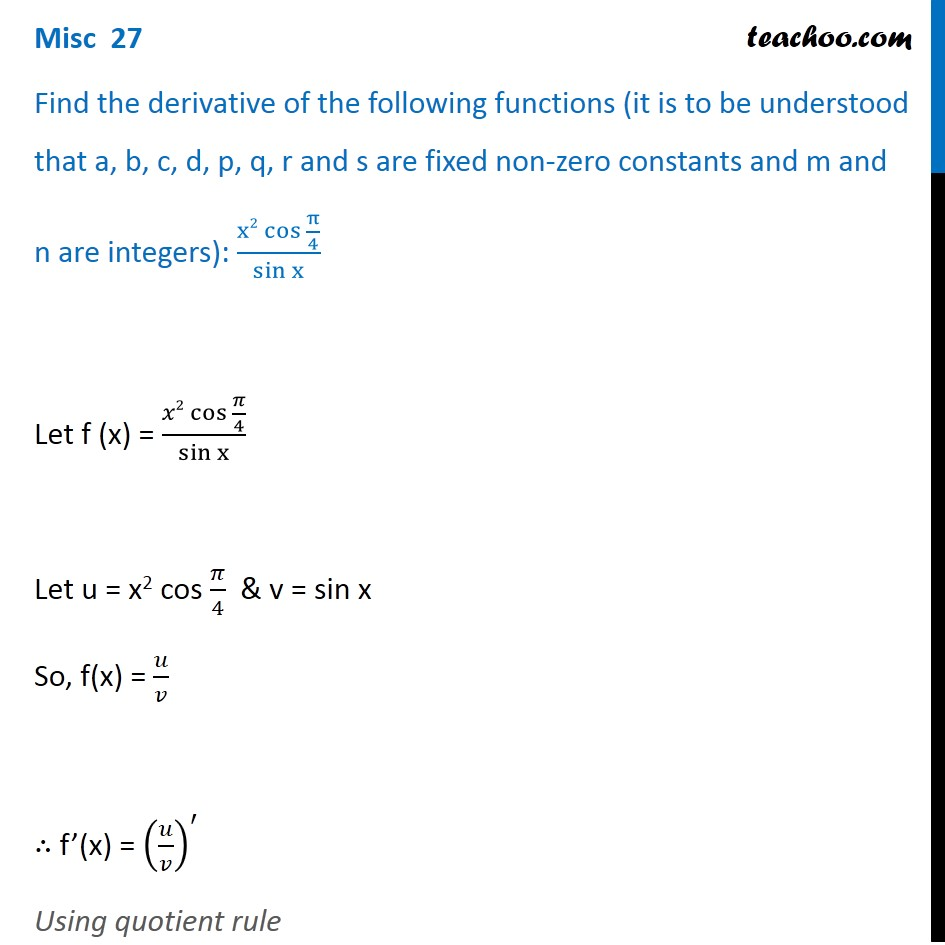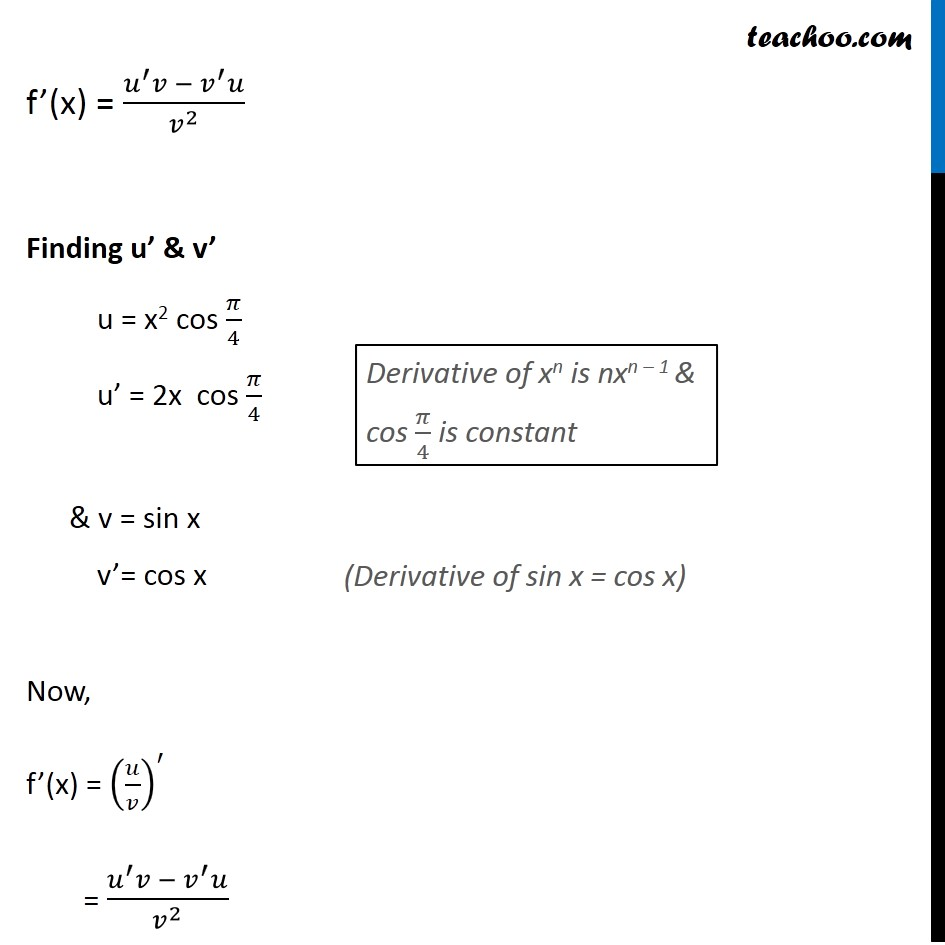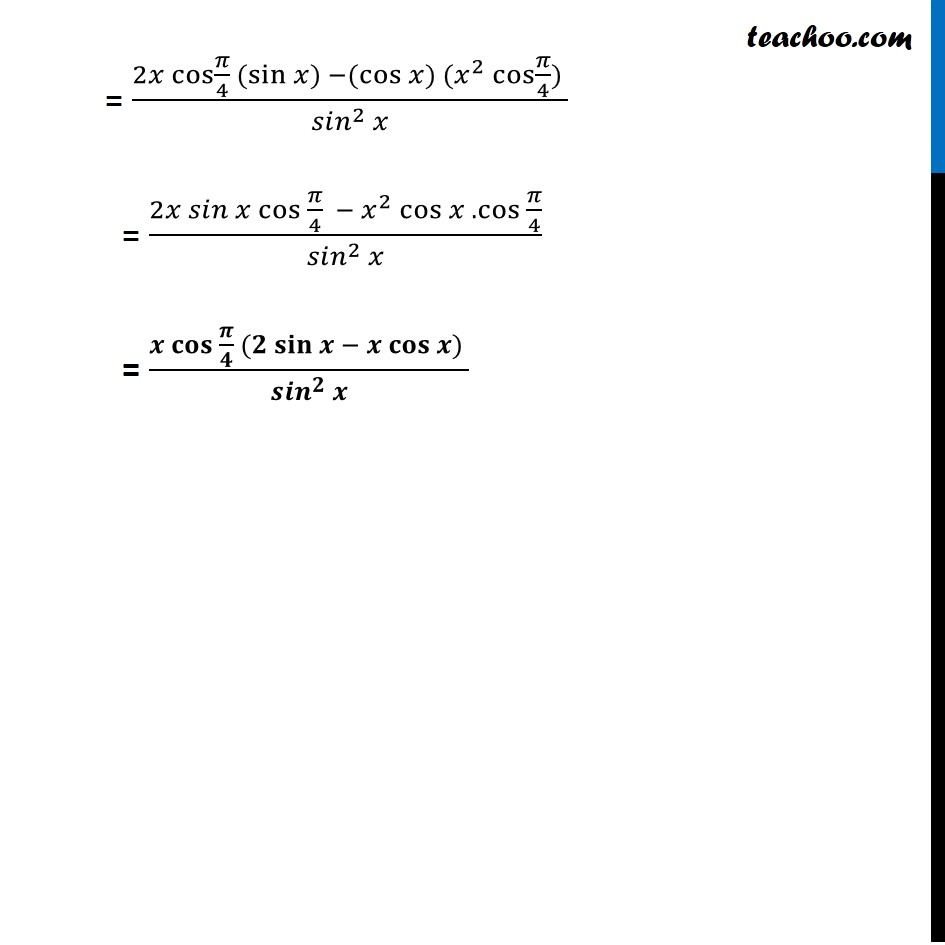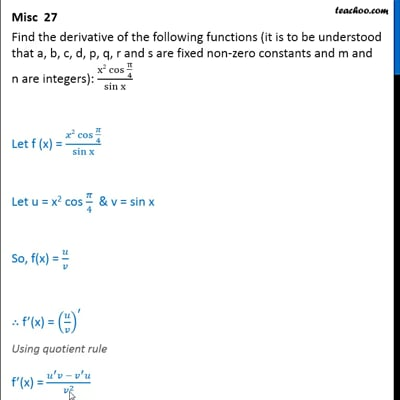Derivatives by formula - sin & cos

Chapter 13 Class 11 Limits and Derivatives
Concept wiseThis video is only available for Teachoo black users

Introducing your new favourite teacher - Teachoo Black, at only ₹83 per month

### Transcript

Misc 27 Find the derivative of the following functions (it is to be understood that a, b, c, d, p, q, r and s are fixed non-zero constants and m and n are integers): (x2 〖cos 〗⁡〖π/4〗)/sin⁡x Let f (x) = (𝑥2 〖cos 〗⁡〖𝜋/4〗)/(sin x) Let u = x2 cos 𝜋/4 & v = sin x So, f(x) = 𝑢/𝑣 ∴ f’(x) = (𝑢/𝑣)^′ Using quotient rule f’(x) = (𝑢^′ 𝑣 −〖 𝑣〗^′ 𝑢)/𝑣^2 Finding u’ & v’ u = x2 cos 𝜋/4 u’ = 2x cos 𝜋/4 & v = sin x v’= cos x Now, f’(x) = (𝑢/𝑣)^′ = (𝑢^′ 𝑣 −〖 𝑣〗^′ 𝑢)/𝑣^2 Derivative of xn is nxn – 1 & cos 𝜋/4 is constant (Derivative of sin x = cos x) = (2𝑥 cos⁡〖𝜋/4〗 (sin⁡〖𝑥)〗 −(cos⁡〖𝑥) (𝑥^2 cos⁡〖𝜋/4) 〗 〗)/(〖𝑠𝑖𝑛〗^2 𝑥) = (2𝑥 〖𝑠𝑖𝑛 𝑥 cos 〗⁡〖𝜋/4〗 − 𝑥^2 cos⁡〖𝑥 .〖cos 〗⁡〖𝜋/4〗 〗)/(〖𝑠𝑖𝑛〗^2 𝑥) = (𝒙 〖𝐜𝐨𝐬 〗⁡〖𝝅/𝟒〗 (𝟐 𝐬𝐢𝐧⁡〖𝒙 − 𝒙 𝐜𝐨𝐬⁡〖𝒙) 〗 〗)/(〖𝒔𝒊𝒏〗^𝟐 𝒙)